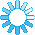Algorithms in C#Welcome to Algorithms & Artificial Intelligence section of C# Corner. In this section, you will find various Algorithms and Artificial Intelligence related source code samples, articles, tutorials, and tips.## RECENT POSTS

• ### Word Search Using Backtracking Algorithm

In this article, you will learn how to search for Word in a N X N Board.
Aug 25, 2020

Jul 24, 2020
• ### Solving the N-Queens Problem

In this blog, you will learn about the N-Queens problem.
Jul 09, 2020
• ### String Algorithm - Program To Validate Given String A Palindrome Permutation

This article describes how to build an algorithm to check if the given string is a permutation of palindrome string.
Sep 13, 2019

Sep 10, 2019
• ### String Algorithm - Validating If String Conversion Requires More Than One Edits

This article describes the algorithm to validate how many edits are required to convert one string into another string
Sep 03, 2019
• ### String Algorithm - Validating If String Has Unique Characters

This article describes an algorithm program to validate if the given string contains unique characters.
Aug 22, 2019
• ### Let's Do Algorithms - Part One

In this series, I will be sharing some algorithm problems and my solutions to the algorithm. The solutions are written in C#.
Aug 21, 2019
• ### C# Data Structures - Stack

In this blog, you will learn about the Stack data structure used in C#.
Aug 18, 2019
• ### String Algorithm - Check String Permutation

This article describes the algorithm to validate if two given strings are permutation combination of each other.
Aug 07, 2019
• ### Merge Sort Algorithm

In this video, the Merge sort explained in plain English. You will learn how the Merge sort works, how to implement it, and how to program with it.
Jul 08, 2019
• ### Merge Sort In C#

This blog explains how to implement a Merge Sort in C# with code example.
Apr 06, 2019
• ### Calculate Fibonacci Series In Various Ways Using C#

This article provides various ways to calculate the Fibonacci series including iterative and recursive approaches, It also exlains how to calculate Nth Fibonacci number.
Mar 31, 2019
• ### C# Artificial Intelligence (AI) Programming: A Basic Object Oriented (OOP) Framework for Neural N...

A Neural Network is an Artificial Intelligence (AI) methodology that attempts to mimic the behavior of the neurons in our brains. In this article, we’ll be building a basic framework for AI Neural ...
Mar 28, 2019
• ### Packing Two Dimensional Rectangular Elements At Orthogonal Table

Here is a program made for two dimensional rectangular elements at orthogonal table in sequence along the X axis of the table, with horizontal orientation exclusively.
Jun 06, 2018
• ### Implement Symmetric And Asymmetric Cryptography Algorithms With C#

How to encrypt and decrypt data using Symmetric and Asymmetric algorithms using C# Programming language
Dec 09, 2017
• ### Binary Search Implementation Using C#

This blog talks about the Binary Search Implementation using both the iterative and the recursive approaches in C#.
Nov 30, 2017
• ### Searching Algorithms In C#

This algorithm will perform a sequential search of item in given array. Every element is checked from start to end and if a match is found the index of matched element will be returned, otherwise -...
Sep 02, 2017
• ### Quick Sort Algorithm In C#

In this article, I am going to explain about the Quicksort algorithm.This is a Divide and Conquer algorithm.It picks an element as pivot and partitions the given array around the picked pivot.
Aug 31, 2017
• ### Data Structures and Algorithm (DSA) – Performance, Complexity And Big-O Notation

In this article we will be talking about what performance of an algorithm is and how Complexity is used to measure performance and what Big-O notation is.
Aug 29, 2017
• ### Bubble Sort Algorithm In C#

In this blog, I am going to explain about the Bubble sort algorithm. Bubble sort is one of the most widely used sorting algorithms by the programmers worldwide. It can be applied to any collection ...
Aug 29, 2017
• ### Merge Sort Algorithm In C#

In this blog, I will be discussing Merge sort algorithm.Merge sort is a comparison based sorting algorithm based on the divide and conquer approach.
Aug 28, 2017
• ### Insertion Sort Algorithm In C#

In this article I am going to explain about the Insertion sort algorithm. Insertion Sort is based on the idea of consuming one element from input array in each iteration to find its correct positio...
Aug 23, 2017
• ### Selection Sort Algorithm In C#

In this blog, I am going to explain about the Selection sort algorithm.
Aug 19, 2017
• ### Data Structures And Algorithms - Part Two - A Word About Big-O Notation

• View More